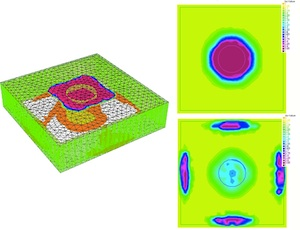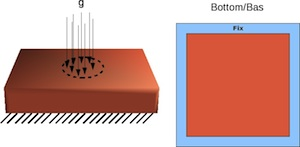Plate Density OptimizationA simple introductory example is to optimize the distribution of elastic material in a thick plate under load in order to minimize the structure's compliance. The algorithm proceeds by a gradient descent on the elastic energy density of the structure. The total amount of material in the design is constrained by a Lagrange multiplier on the descent step. Numerically implementation of this method requires the use of two capabilities of FreeFEM++: the construction of the three-dimensional mesh, and iterative solution of the PDEs of elasticity by the Finite Element Method on this mesh. For simple structures such as thick plates, the mesh is easily constructed using FreeFEM's internal layer meshing tool. Here a mesh is constructed in two dimensions (x,y) and essentially extruded by copying it in the third (z) and tetrahedralizing. The Finite Element Method is implemented using the Conjugate Gradient solver incorporated in FreeFEM++.

The script for this density optimization algorithm in FreeFEM++ can be downloaded here.